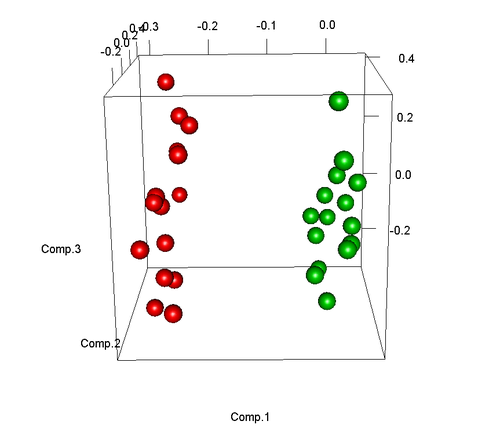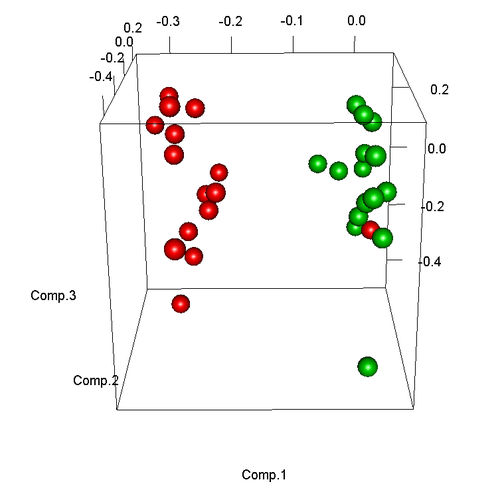# 主成份分析（PCA）在生物芯片样本筛选中的应用及在R语言中的实现

10,444

`chip.dat<-matrix(rnorm(10000*30,mean=0),ncol=30,nrow=10000)`

`colour<-c(rep(2,15),rep(3,15))`

```diff.ind<-sample(1:10000,100)
chip.dat[diff.ind[1:50],1:15]<-rnorm(50*15,mean=2)
chip.dat[diff.ind[51:100],1:15]<-rnorm(50*15,mean=-2)```

`chip.dat.pca<-princomp(chip.dat)`

```library(rgl)```chip.dat<-matrix(rnorm(10000*30,mean=0),ncol=30,nrow=10000)
chip.dat[diff.ind[1:50],1:14]<-rnorm(50*14,mean=2)
chip.dat[diff.ind[51:100],1:14]<-rnorm(50*14,mean=-2)```

```chip.dat.pca<-princomp(chip.dat)